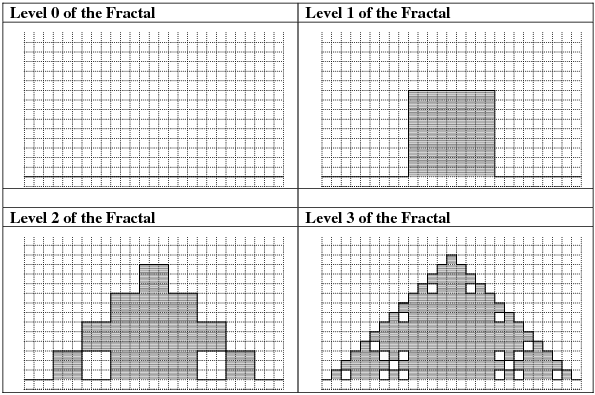## Problem J5: Fractals

A fractal is a geometric shape where the pattern of the whole shape is self-replicating at each subsection of the shape. A simple 'block fractal' is shown below. At each stage of the fractal growth, every straight line in the fractal is divided into three equal parts. The first and last sections stay straight; the middle section contains a square 'bump which has the same height as the width of the middle section. (You will want to consider the four orientation of a line segment within the fractals. Depending upon which line segment is currently being generated, the bump may protrude up, down, left or right.)Suppose this fractal is drawn on a Cartesian plane, where (0, 0) is at the bottom left corner. Assume that in the example above, the bottom left point of the fractal is at (0, 1) and the bottom right point of the fractal is at (27, 1). For example, the top of the Level 3 fractal is a line from (13, 14) to (14, 14).

Write a program that will keep track of the integer coordinate points of the lines in a similar 'block fractal' with its bottom left corner at (0,1). The program will accept three integers as input: the level of the fractal, the width of the fractal, and a x-coordinate. You may assume that the width of the fractal will be some power of three, and that it will be large enough so that every corner of fractal will fall on an integer intersection in the Cartesian plane. The width will never be more than 81. The x-coordinate, x, will be in the range 0 - width(inclusive). Your program should output the y-coordinate value(s), y, where lines of fractal intersect the point(x, y).

You may draw a graphic representation of the fractal for debugging (and interest). However, test cases may ask you to define fractals that are too large to fit on a single screen.

`3 27 5`

`4 5 6`

### Sample Input 2

`3 27 18`

### Sample Output 2

`1 2 3 4 7 8 9 10`

### Sample Input 3

`2 27 19`

`1 4 7`

### Sample Input 4

`4 81 38`

### Sample Output 4

`37 38 39`

Point Value: 10
Time Limit: 2.00s
Memory Limit: 16M